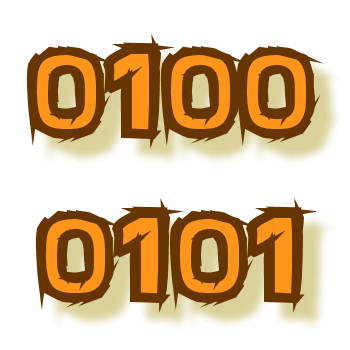Games
Problems
Go Pro!Emmanuel from Nigeria asks, "How are letters converted into binary codes?"

Well, Emmanuel, I'm guessing you came here from our Binary Coding Page. If so, you ask an excellent question, because the subject of how we convert letters is not really explained there.

The big question is, "How do you convert a letter to a number?" Because if you can convert the letter to a number, then you can use the information on our base conversion page to convert that number into binary. So how does the computer do the conversion from letter to number?

There is a standard listing called the "ASCII Character Set," in which every character used on a computer's keyboard is assigned a number. There are a lot of these characters, because there isn't just one for every key on your keyboard - there's also one for each key with the SHIFT key pressed. The ASCII character set has room for 256 characters, numbered from 0 to 255.

You might wonder, "Why 256?" and the answer is, because 256 = 28, which means anything less than 256 can be written as 8 binary bits (place values). Each character needs to have the same number of binary bits - otherwise nobody would know where one character ends and the next one starts. So even though the number 15 only needs four bits to be written in binary (1111two) in order to make sure all the numbers have the same length, the computer would write it as 0000 1111two. We put a space between every four digts, for the same reason that we do commas in base ten - it helps us read long strings of digits more easily.

Okay, so with that out of the way, now we just need to know what letters are represented by what number. Here's a quick reference for you:

A = 65
B = 66
C = 67
...
X = 88
Y = 89
Z = 90

Remember I mentioned that the ASCII Codes account for the shift key? That means that lower case letters have a different number assigned than upper case letters:

a = 97
b = 98
c = 99
...
x = 120
y = 121
z = 122

Now, there are a couple things you might have wondered about, like "What comes before 65?" and "Why is there a gap between the upper and lower case numbers?"

The answer to the first question is, there are other characters in those gaps - numbers, punctuation, special control characters (like the Backspace, Enter, Delete, etc).

The reason there's a gap between the upper case and lower case alphabets is that it makes "a" 32 more than "A." That is very convenient because 32 is a power of 2 (25), so changing between upper and lower case means changing just one bit:

A = 0100 0001
a = 0110 0001

So if you're using the computer's ASCII character set, and you wanted to convert "Hello" into binary, you would look up each letter in the ASCII chart:

H = 72 = 0100 1000two
e = 101 = 0110 0101two
l = 108 = 0110 1100two
l = 108 = 0110 1100two
o = 111 = 0110 1111two

So the entire word "Hello" is:

0100 1000 0110 0101 0110 1100 0110 1100 0110 1111two.

BUT...you don't have to use the ASCII conversion; you could create your own way of converting letters to numbers. Why would you want to do that? Well, you probably wouldn't, unless you wanted to conserve space, and you didn't care about anything except the basic upper case alphabet.

You see, if all you cared about was the upper case letters (and maybe a space), then you could do a conversion like this:

SPACE = 0
A = 1
B = 2
C = 3
...
X = 24
Y = 25
Z = 26

Why would you want to do that? Because now your biggest number you need to encode is 26, which is less than 25. That means that you only need five digits to write each number instead of eight! So your encoded message will take up 5/8 as much space. You'll save about 38% of the space on the page.

Since you have to have space in your table for 32 characters, and you've only used up to 26, you might as well use the other ones. Maybe include some punctuation?

COMMA = 27
PERIOD = 28
QUESTION MARK = 29
DASH = 30
DOLLAR SIGN = 31

Or you could create a table with 64 characters, which would either let you put in a lot more punctuation, or the numbers, or the lower case alphabet. But now you're using six binary digits per character, so you're not saving as much space.

Or you could completely jumble your character chart, which makes it a harder for other people to decode:

A = 17
B = 3
C = 25
etc...

But if you really want to make a coded message, there are much better ways to do it, so everyone just sticks with the standard ASCII codes in order to keep things simple.

A couple more things:

1. The ASCII chart is available online; just go to google and search for "ASCII codes" and you'll get the entire list!
2. ASCII stands for "American Standard Code for Information Interchange"

Thanks for asking, Emmanuel. I probably gave you much more information than you were expecting, but I hope you found it both interesting and helpful!

Professor PuzzlerLike us on Facebook to get updates about new resources